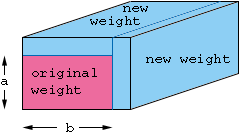My name is Lee Millard and I am currently attending Conestoga College in Ontario, Canada. And I need help with a problem. You have a great site and your help would be greatly appreciated! A weight of dimension a, b is to have its weight increased by w% by bolting plates of the same material along the top and one side (but not at both ends). The top plate and side plate have the thickness, x. Find the thickness of the plates for a given weight increase of w%. I know that you use the areas to find the weight increase. The variable x is easy to find if the original weight is square, but what if it isn't? There's got to be some derivative equation or something for x. Uh, I am lost! Please help me! Thanks, Lee Millard Hi Lee, You have the right idea, but I don't think that it matters if the original weight is a square. Since the plates you added are of thickness x the dimensions of the end of the modilied block are a+x and b+x (a and b are known dimensions, it is only x that is unknown). Thus the area of the end is (a+x)(b+x). This is to be a w% increase in area so (a+x)(b+x) = ab + (w/100) ab. Now expand the left side, collect everything together as a quadratic in x and solve.Cheers, Penny Go to Math Central